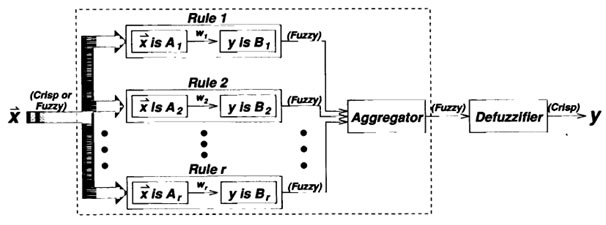### Introduction

The fuzzy inference system is a popular computing framework based on the concepts of fuzzy set theory, fuzzy if-then rules, and fuzzy reasoning. It has found successful applications in a wide variety of fields, such as automatic control, data classification, decision analysis, expert systems time series prediction, robotics, and pattern recognition. Because of its multidisciplinary nature, the fuzzy inference system is known by numerous other names, such as fuzzy-rule-based system, fuzzy expert system, fuzzy model, fuzzy associative memory, fuzzy logic controller, and simply (and ambiguously) fuzzy system.

The basic structure of a fuzzy inference system consists of three conceptual components:

• A rule base, which contains a selection of fuzzy rules;
• A database (or dictionary), which defines the membership functions used in the fuzzy rules;
• And a reasoning mechanism, which performs the inference procedure upon the rules and given facts to derive a reasonable output or conclusion.

Note that the basic fuzzy inference system can take either fuzzy inputs or crisp inputs (which are viewed as fuzzy singletons), but the outputs it produces are almost always fuzzy sets. Sometimes it is necessary to have a crisp output, especially in a situation where a fuzzy inference system is used as a controller. Therefore, we need a method of defuzzification to extract a crisp value that best represents a fuzzy set. A fuzzy inference system with a crisp output is shown in Figure 1, where the dashed line indicates a basic fuzzy inference system with fuzzy output and the defuzzification block serves the purpose of transforming an output fuzzy set into a crisp single value.Figure 1: Block diagram for a fuzzy inference system

With crisp inputs and outputs, a fuzzy inference system implements a nonlinear mapping from its inputs space to output space. This mapping is accomplished by a number of fuzzy if-then rules, each of which describes the local behaviour of the mapping. In particular, the antecedent of a rule defines a fuzzy region in the input space, while the consequent specifies the output in the fuzzy region. The most frequently used fuzzy inference systems are:

### References & Resources

• Book, Neuro-fuzzy and soft computing a computational approach to learning and machine intelligence